Main Content

## Using RF Measurement Testbench

Use the RF Measurement testbench to verify the cumulative gain, noise figure, and nonlinearity (IP3) values of an RF-to-RF system. To use the testbench, create a system in the RF Budget Analyzer app and click `Export` > ```Export to Measurement Testbench```.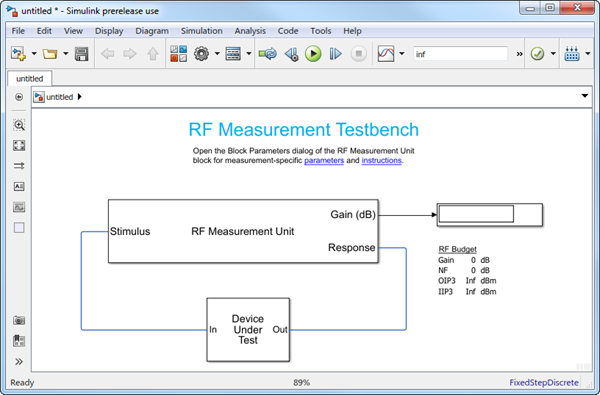The testbench is made up of two subsystems:

• `RF Measurement Unit`

• `Device Under Test`

The testbench display shows the verified output values of gain, NF (noise figure), and IP3 (third-order intercept).

### Device Under Test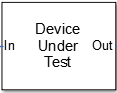The Device Under Test subsystem contains the RF system exported from the app.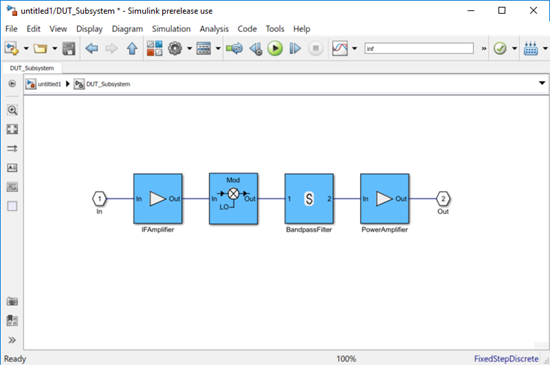### RF Measurement Unit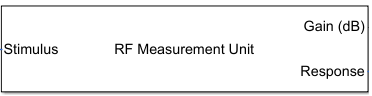The RF Measurement Unit subsystem consists of a Simulink Controller and RF Blockset Circuit Envelope interface. The RF Blockset interface is used as input and output from the DUT.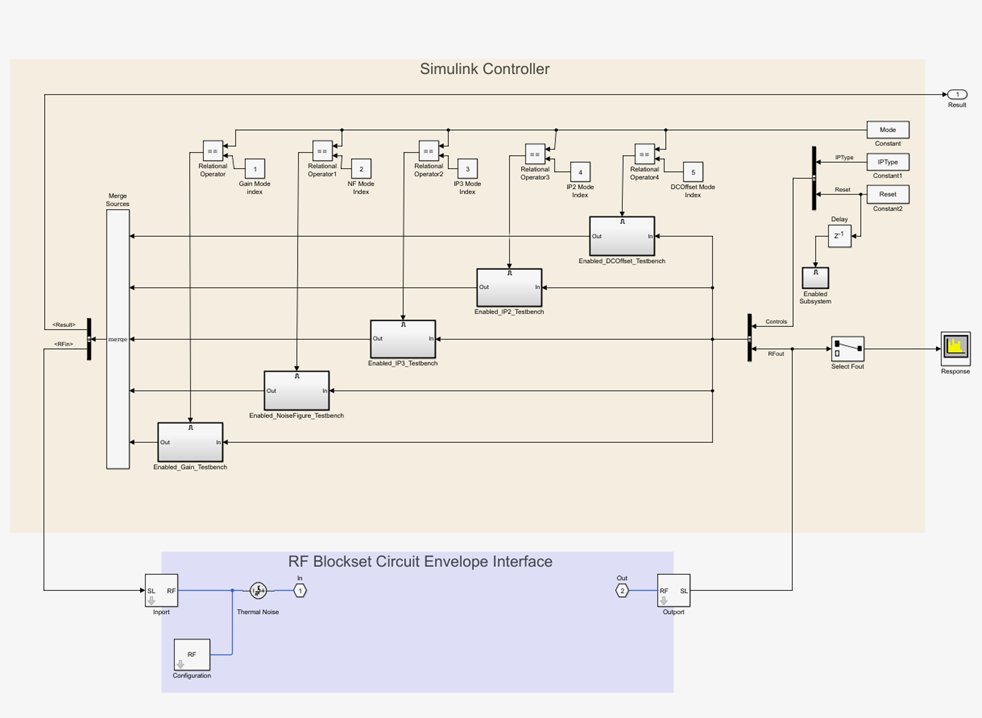### RF Measurement Unit Parameters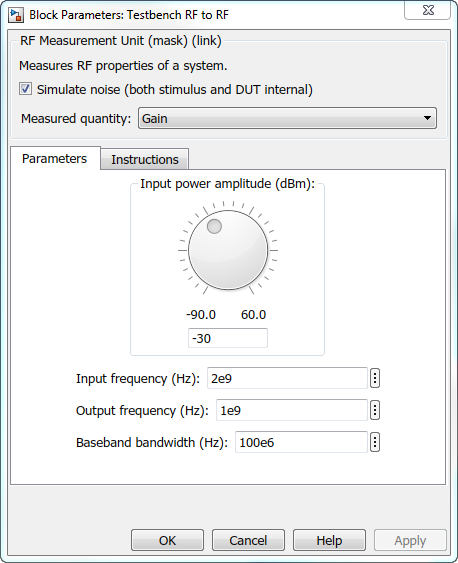Double-click the RF Measurement Unit subsystem block to open the parameters.

• Simulate noise (both stimulus and DUT internal) — Select this check box to enable noise modeling in the stimulus signal entering the DUT and inside the DUT.

• Measured quantity — Choose the quantity you want to verify from:

• `Gain` – Measure the transducer gain of the converter, assuming a load of 50 ohm. If you choose only `I` or only `Q` from Response branch, you see only half the value of the measured gain.

• `NF` – Measure the noise figure value at the output of the converter.

• `IP3` – Measure the output or input third-order intercept (IP3).

• `IP2` – Measure the output or input second-order intercept (IP2).

• `DC Offset` – Measure the DC level interference centered on the desired signal due to LO leakage mixing with input signal.

The contents in the Instructions tab changes according to the Measured quantity.

• IP Type — Choose the type of intercept points (IP) to measure: `Output referred` or ```Input referred```.

By default, the testbench measures `Output referred`. This option is available when you set the Measured quantity to `IP2` or `IP3`.

#### Parameters Tab

• Input power amplitude (dBm) — Input power to the DUT. You can change the input power by manually specifying or by turning the knob. When measuring ```DC Offset```, this input field is Input RMS voltage (dBmV), because the Offset is measured in voltage units. The specified voltage represents the voltage falling on the input ports of the DUT.

• Input frequency (Hz) — Carrier frequency of the DUT.

• Output frequency (Hz) — Output frequency of the DUT.

• Baseband bandwidth (Hz) — Bandwidth of the input signal.

• Ratio of test tone frequency to baseband bandwidth — Position of the test tones used for IP3 measurements. By default, the value is `1/8`.

This option is available when you set the Measured quantity to `IP2`, `IP3`, or `DC Offset`.

#### Instructions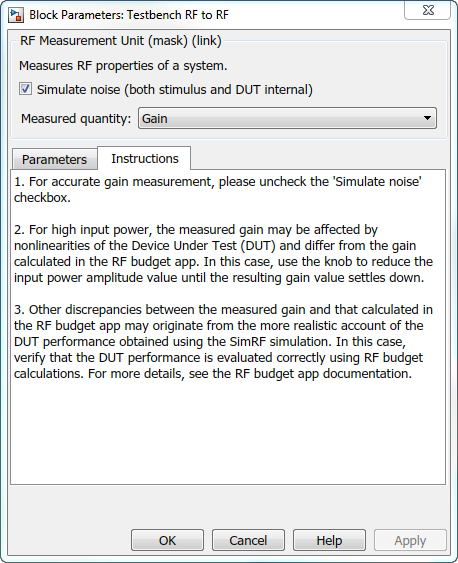Instructions for Gain Verification

• Clear Simulate noise (both stimulus and DUT) for accurate gain verification. Select the check box for account for noise.

• Change the Input power amplitude (dBm) or turn the knob to reduce the input power amplitude. For high input power, nonlinearities in the DUT can affect the gain measurements.

Instructions for NF Verification

• The testbench verifies the spot NF calculated. This calculation assumes a frequency-independent system within a given bandwidth. To simulate a frequency-independent system and calculate the correct NF value, reduce the baseband bandwidth until this condition is fulfilled. In common RF systems, the bandwidth should be reduced below 1 kHz for NF testing.

• Change Input power amplitude (dBm) or turn the knob to reduce or increase the input power amplitude. For high input power, nonlinearities in the DUT can affect the NF measurements. For low input power, the signal is too close or below the noise floor of the system. As a result, the NF fails to converge.

Instructions for OIP3 and IIP3 Verification

• Clear Simulate noise (both stimulus and DUT) for accurate OIP3 and IIP3 verification.

• Change Input power amplitude (dBm) or turn the knob to reduce the input power amplitude. For high input power, higher-order nonlinearities in the DUT can affect the OIP3 and IIP3 measurements.

For all measurement verifications using the testbench, you cannot correct result discrepancies using the RF Budget Analyzer app. The RF Blockset testbench provides true RF circuit simulation that incorporates RF phenomena including saturation and interaction between multiple tones and harmonics in nonlinear devices. These RF phenomena are not yet incorporated in RF Budget Analyzer, leading to some differences in the values between the testbench and the app.

Instructions for DC Offset Measurement

• Clear Simulate noise (both stimulus and DUT) for accurate DC offset measurement.

• Correct calculation of the DC offset assumes a frequency-independent system in the frequencies surrounding the test tones. Reduce the frequency separation between the test tones or reduce the baseband bandwidth until this condition is fulfilled. In common RF systems, the bandwidth is reduced below `1 KHz` for DC offset testing.

• . Change Input RMS voltage amplitude (dBmV) or turn the knob to reduce the input RMS voltage amplitude. For high input RMS voltage, higher-order nonlinearities in the DUT can affect the DC offset measurements

## Support

#### Model RF Power Amplifiers and Increase Transmitter Linearity with DPD Using MATLAB

Download white paper